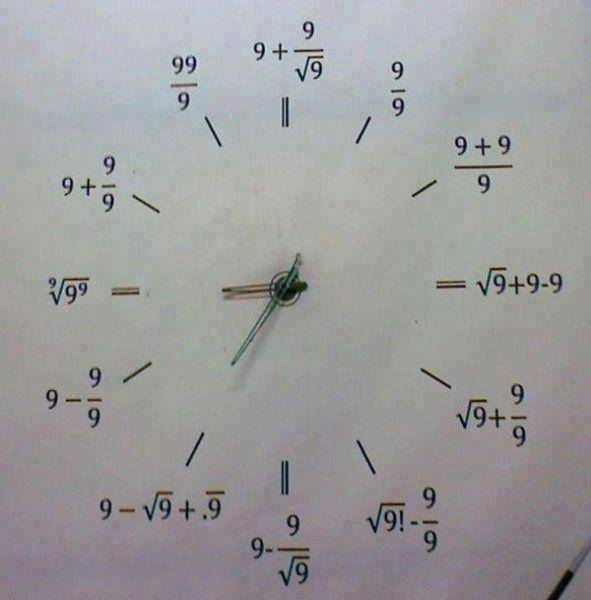# Exploring Oddities of Integer Relationships

• Simon Bridge

#### Simon Bridge

Homework Helper
I come across some odd stuff online...... OK there's a typo for the 5 ... should be ##\small [\sqrt{9}]!-(9/9)## and the one for 7 looks a bit forced...
What I'm wondering is if there are other sets that do something like this ... i.e. so for a given integer Z, we can find another integer z<Z so that the integers ≤Z can be represented using the same n (integer) instances of z in each case.

It'll probably help of Z is something with lots of divisors ... i.e. Z= oh I dunno... 12.
Or is it just a case of "given sufficient cleverness" - which is to say that there are so many legitimate mathematical operations that it is always possible to create this effect?

Aside:
Millenialists take note: hold the clock upside down and all the numbers are made out of three 6's.

I'm sure there are lists of that somewhere.

1=(X/X)^X
2=(X+X)/X
Those two are always possible.
9 is nice, as you can choose between 9 and 3 via the square root.
With small even numbers, it is tricky to get large odd numbers.